# Mining Optimal Decision Trees from Itemset Lattices KDD

• Slides: 34Mining Optimal Decision Trees from Itemset Lattices KDD’ 07 Presented by Xiaoxi Du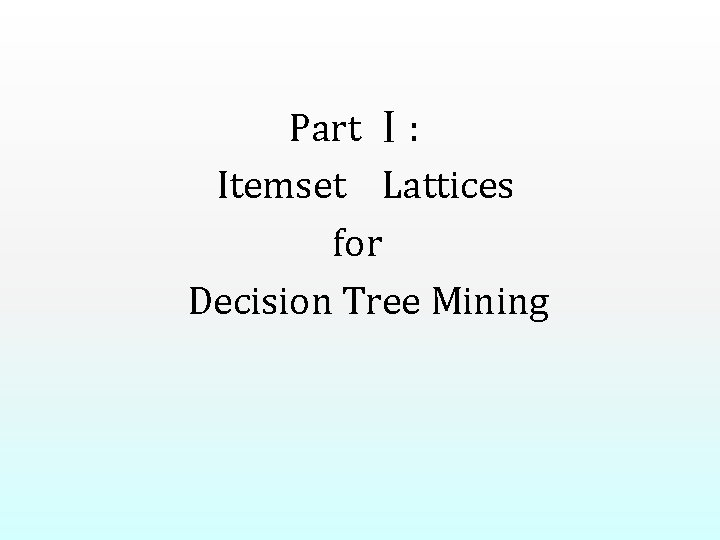Part Ⅰ: Itemset Lattices for Decision Tree Mining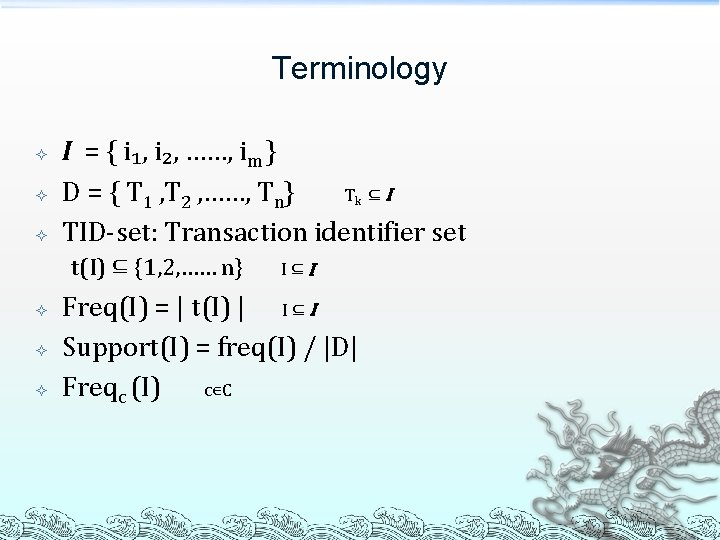Terminology I = { i₁, i₂, ……, im } D = { T 1 , T 2 , ……, Tn} Tk ⊆ I TID-set: Transaction identifier set t(I) ⊆ {1, 2, …… n} I⊆I Freq(I) = | t(I) | I ⊆ I Support(I) = freq(I) / |D| Freqc (I) c∊C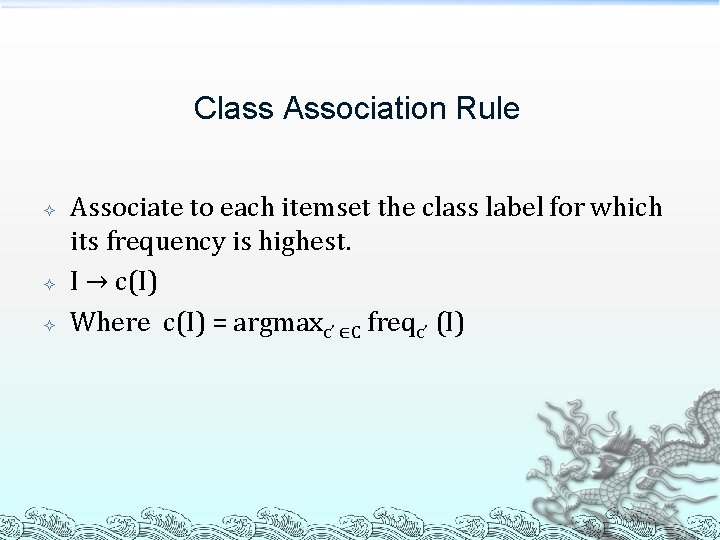Class Association Rule Associate to each itemset the class label for which its frequency is highest. I → c(I) Where c(I) = argmaxc’ ∈C freqc’ (I)The Decision Tree Assume that all tests are boolean; nominal attributes are transformed into boolean attributes by mapping each possible value to a separate attribute. The input of a decision tree is a binary matrix B, where Bij contains the value of attribute i of example j. Observation: let us transform a binary table B into transactional form D such that Tj = {i|Bij =1} ∪ {⇁i|Bij =0}. Then the examples that are sorted down every node of a decision tree for B are characterized by an itemset of items occurring in D.Example: the decision tree B 1 0 C 1 1 {B} Leaves(T ) 1 {⇁BC} Path (T)={∅, {B}, {⇁B, C}, {⇁B, ⇁C}} 0 0 {⇁B⇁C}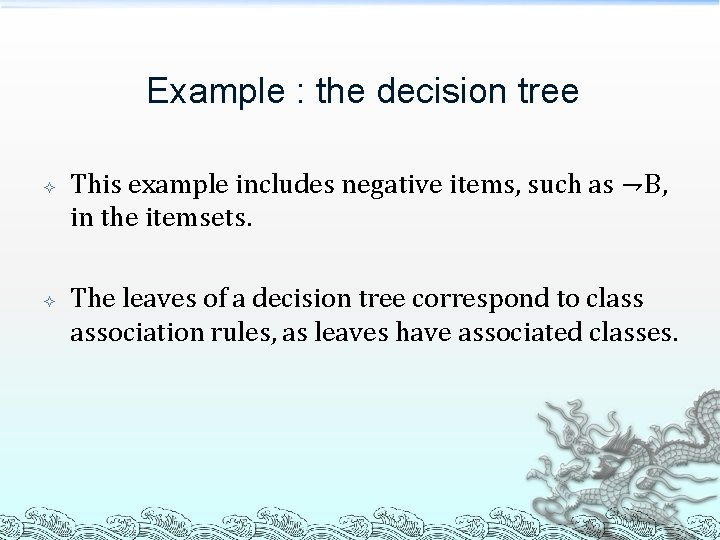Example : the decision tree This example includes negative items, such as ⇁B, in the itemsets. The leaves of a decision tree correspond to class association rules, as leaves have associated classes.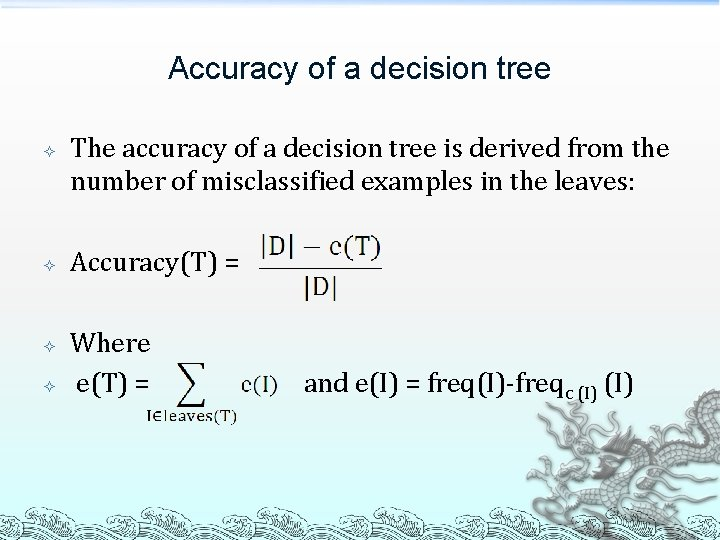Accuracy of a decision tree The accuracy of a decision tree is derived from the number of misclassified examples in the leaves: Accuracy(T) = Where e(T) = and e(I) = freq(I)-freqc (I)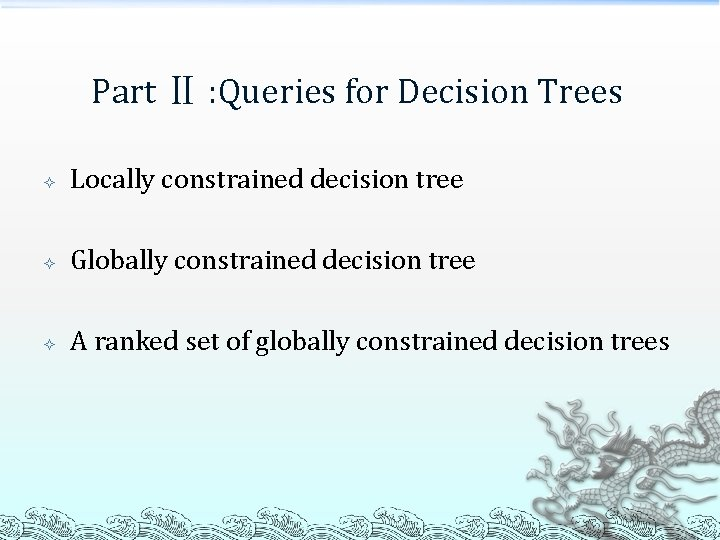Part Ⅱ : Queries for Decision Trees Locally constrained decision tree Globally constrained decision tree A ranked set of globally constrained decision trees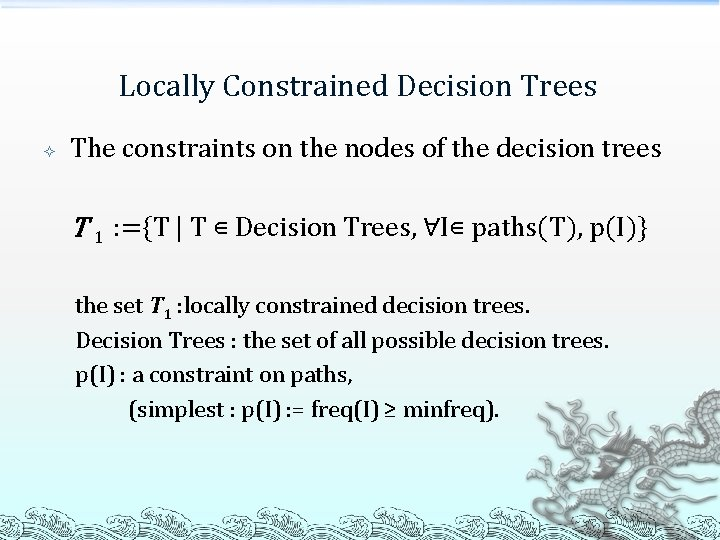Locally Constrained Decision Trees The constraints on the nodes of the decision trees T 1 : ={T | T ∊ Decision Trees, ∀I∊ paths(T), p(I)} the set T 1 : locally constrained decision trees. Decision Trees : the set of all possible decision trees. p(I) : a constraint on paths, (simplest : p(I) : = freq(I) ≥ minfreq).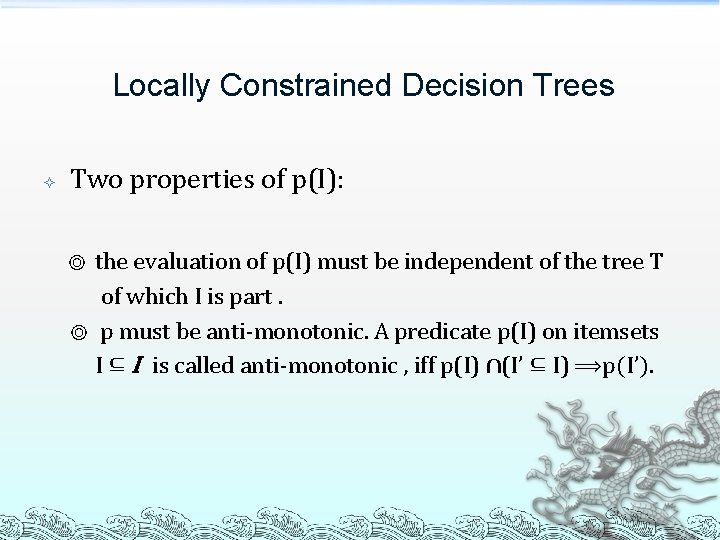Locally Constrained Decision Trees Two properties of p(I): the evaluation of p(I) must be independent of the tree T of which I is part. ◎ p must be anti-monotonic. A predicate p(I) on itemsets I ⊆ I is called anti-monotonic , iff p(I) ∩(I’ ⊆ I) ⟹p(I’). ◎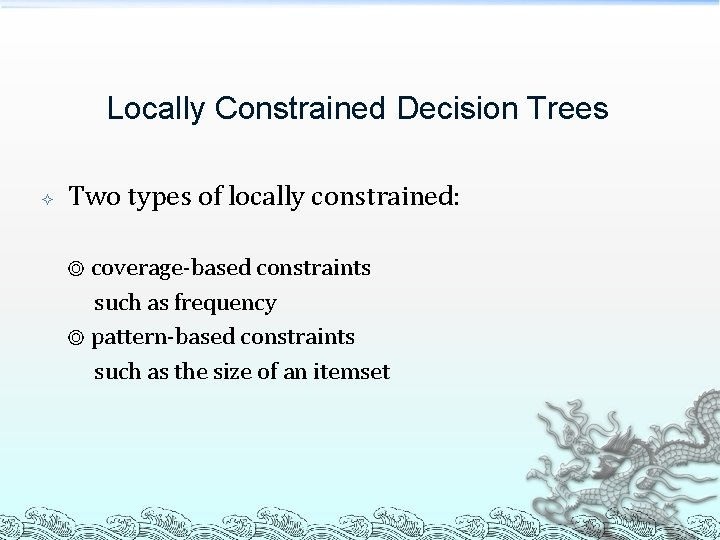Locally Constrained Decision Trees Two types of locally constrained: ◎ coverage-based constraints such as frequency ◎ pattern-based constraints such as the size of an itemsetGlobally Constrained Decision Trees The constraints refer to the tree as a whole Optional part T 2 : = {T | T∊ T 1 , q(T)} the set T 2 : globally constrained decision trees. q(T) : a conjunction of constraints of the form f(T) ≤ ��.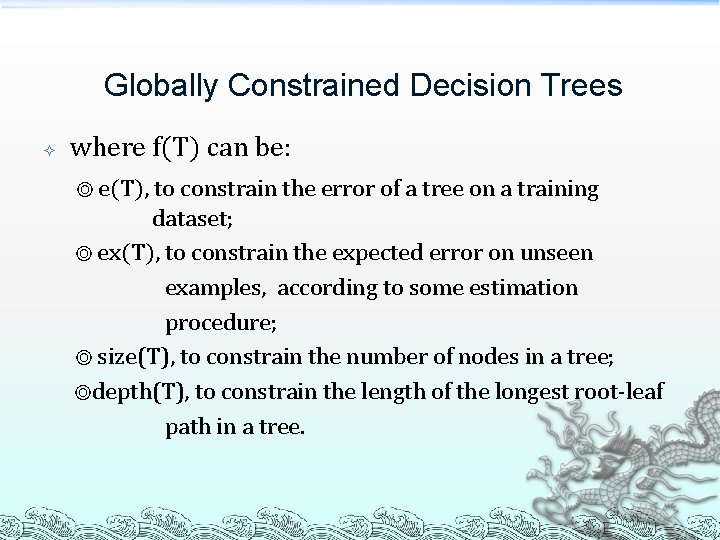Globally Constrained Decision Trees where f(T) can be: ◎ e(T), to constrain the error of a tree on a training dataset; ◎ ex(T), to constrain the expected error on unseen examples, according to some estimation procedure; ◎ size(T), to constrain the number of nodes in a tree; ◎depth(T), to constrain the length of the longest root-leaf path in a tree.A ranked set of globally constrained decision trees Preference for a tree in the set T 2 Mandatory output argmin. T∊T₂ [r 1 (T), r 2 (T), ……, rn (T)] r(T) =[r 1 (T), r 2 (T), ……, rn (T)] : a ranked set of globally constrained decision trees; ri ∊ {e, ex, size, depth}. if depth or size before e or ex, then q must contain an atom depth(T) ≤ maxdepth or size(T) ≤ maxsize.Part Ⅲ: The DL 8 Algorithm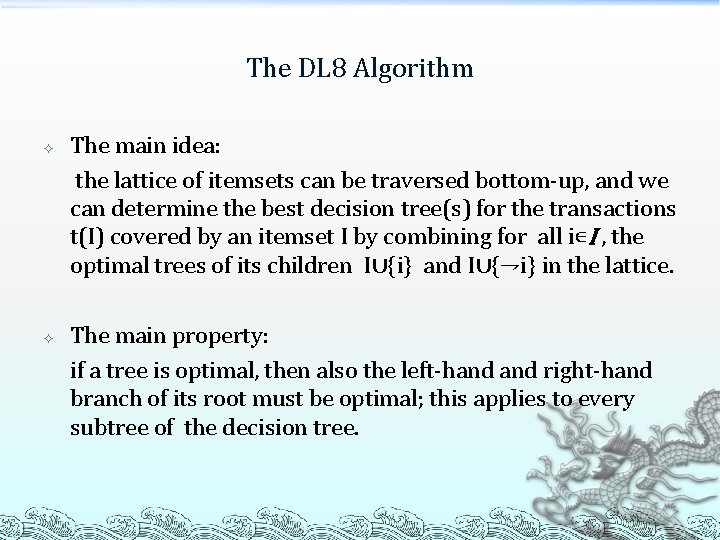The DL 8 Algorithm The main idea: the lattice of itemsets can be traversed bottom-up, and we can determine the best decision tree(s) for the transactions t(I) covered by an itemset I by combining for all i∊I , the optimal trees of its children I∪{i} and I∪{⇁i} in the lattice. The main property: if a tree is optimal, then also the left-hand right-hand branch of its root must be optimal; this applies to every subtree of the decision tree.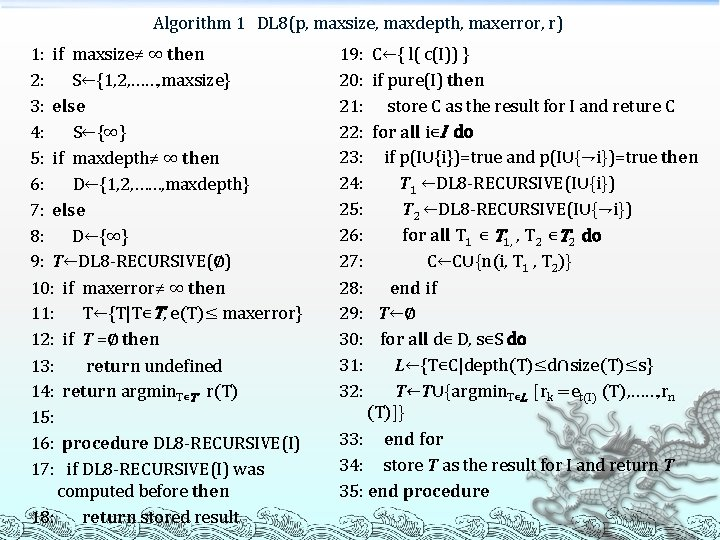Algorithm 1 DL 8(p, maxsize, maxdepth, maxerror, r) 1: if maxsize≠ ∞ then 2: S←{1, 2, ……, maxsize} 3: else 4: S←{∞} 5: if maxdepth≠ ∞ then 6: D←{1, 2, ……, maxdepth} 7: else 8: D←{∞} 9: T←DL 8 -RECURSIVE(∅) 10: if maxerror≠ ∞ then 11: T←{T|T∊T, e(T)≤ maxerror} 12: if T =∅ then 13: return undefined 14: return argmin. T∊T r(T) 15: 16: procedure DL 8 -RECURSIVE(I) 17: if DL 8 -RECURSIVE(I) was computed before then 18: return stored result 19: 20: 21: 22: 23: 24: 25: 26: 27: 28: 29: 30: 31: 32: C←{ l( c(I)) } if pure(I) then store C as the result for I and reture C for all i∊I do if p(I∪{i})=true and p(I∪{⇁i})=true then T 1 ←DL 8 -RECURSIVE(I∪{i}) T 2 ←DL 8 -RECURSIVE(I∪{⇁i}) for all T 1 ∊ T 1, , T 2 ∊T 2 do C←C∪{n(i, T 1 , T 2)} end if T←∅ for all d∊ D, s∊S do L←{T∊C|depth(T)≤d∩size(T)≤s} T←T∪{argmin. T∊L [rk =et(I) (T), ……, rn (T)]} 33: end for 34: store T as the result for I and return T 35: end procedureThe DL 8 Algorithm Parameters DL 8(p, maxsize, maxdepth, maxerror, r) where, p: the local constraint ; r: the ranking function; maxsize, maxdepth, maxerror : the global constraints; (each global constraints is passed in a separate parameter; global constraints that are not specified, are assumed to be set to ∞)The DL 8 Algorithm Line 1 -8: the valid ranges of sizes and depths are computed here if a size or depth constraint was specified. Line 11: for each depth and size satisfying the constraints DL 8 -RECURSIVE finds the most accurate tree possible. Some of the accuracies might be too low for the given constraint, and are removed from consideration. Line 19: a candidate decision tree for classifying the examples t(I) consists of a single leaf.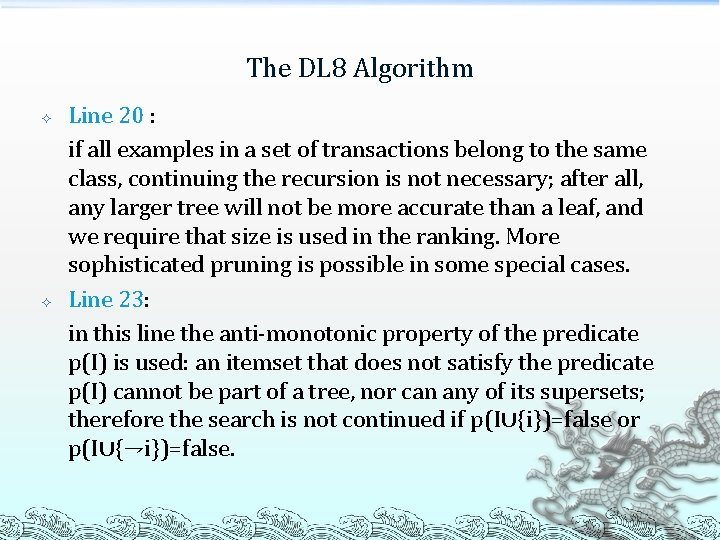The DL 8 Algorithm Line 20 : if all examples in a set of transactions belong to the same class, continuing the recursion is not necessary; after all, any larger tree will not be more accurate than a leaf, and we require that size is used in the ranking. More sophisticated pruning is possible in some special cases. Line 23: in this line the anti-monotonic property of the predicate p(I) is used: an itemset that does not satisfy the predicate p(I) cannot be part of a tree, nor can any of its supersets; therefore the search is not continued if p(I∪{i})=false or p(I∪{⇁i})=false.The DL 8 Algorithm Line 22 -33: these lines make sure that each tree that should be part of the output T , is indeed returned. We can prove this by induction. Assume that for the set of transactions t(I), tree T should be part of T as it is the most accurate tree that is smaller than s and shallower than d for some s∊S and d∊D; assume T is not a leaf, and contains test in the root. Then T must have a left-hand branch. T 1 and a right-hand branch T 2. Tree T 1 must be the most accurate tree that can be constructed for t(I∪{⇁i}) under depth and size constraints. We can inductively assume that trees with these constraints are found by DL 8 -RECURSIVE(I∪{i}) and DL 8 RECURSIVE(I∪{⇁i}) as size(T 1), size(T 2)≤maxsize and depth(T 1), depth(T 2)≤maxdepth. Consequently T(or a tree with the same properties) must be among the trees found by combining results from the two recursive procedure calls in line 27.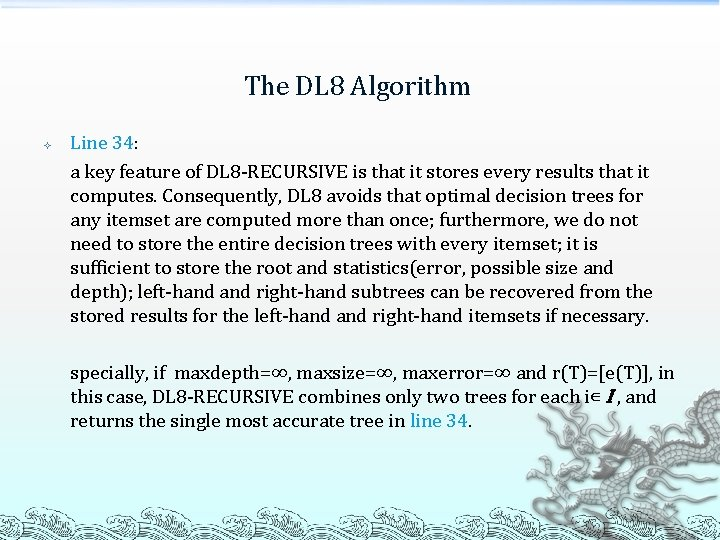The DL 8 Algorithm Line 34: a key feature of DL 8 -RECURSIVE is that it stores every results that it computes. Consequently, DL 8 avoids that optimal decision trees for any itemset are computed more than once; furthermore, we do not need to store the entire decision trees with every itemset; it is sufficient to store the root and statistics(error, possible size and depth); left-hand right-hand subtrees can be recovered from the stored results for the left-hand right-hand itemsets if necessary. specially, if maxdepth=∞, maxsize=∞, maxerror=∞ and r(T)=[e(T)], in this case, DL 8 -RECURSIVE combines only two trees for each i∊ I , and returns the single most accurate tree in line 34.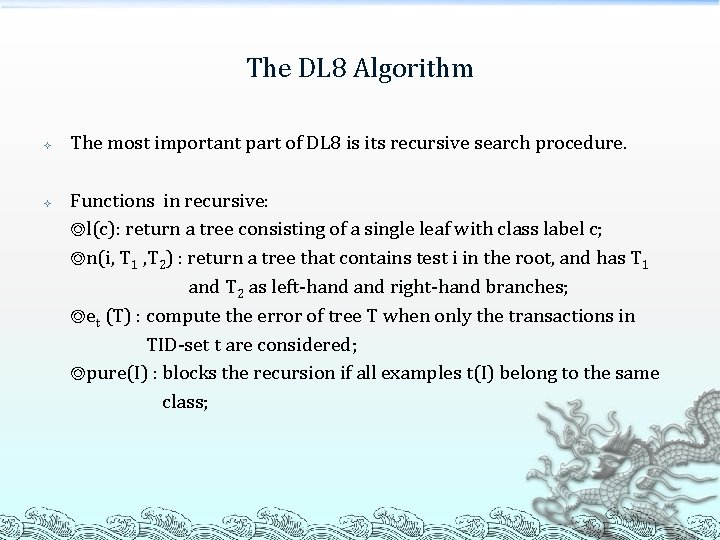The DL 8 Algorithm The most important part of DL 8 is its recursive search procedure. Functions in recursive: ◎l(c): return a tree consisting of a single leaf with class label c; ◎n(i, T 1 , T 2) : return a tree that contains test i in the root, and has T 1 and T 2 as left-hand right-hand branches; ◎et (T) : compute the error of tree T when only the transactions in TID-set t are considered; ◎pure(I) : blocks the recursion if all examples t(I) belong to the same class;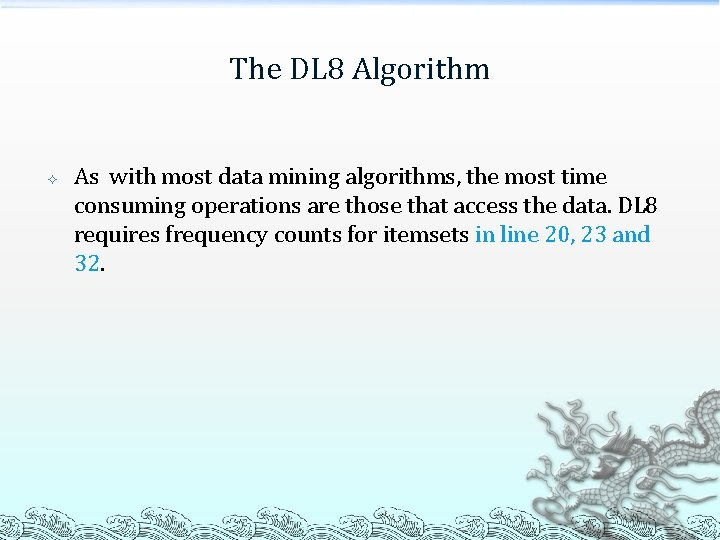The DL 8 Algorithm As with most data mining algorithms, the most time consuming operations are those that access the data. DL 8 requires frequency counts for itemsets in line 20, 23 and 32.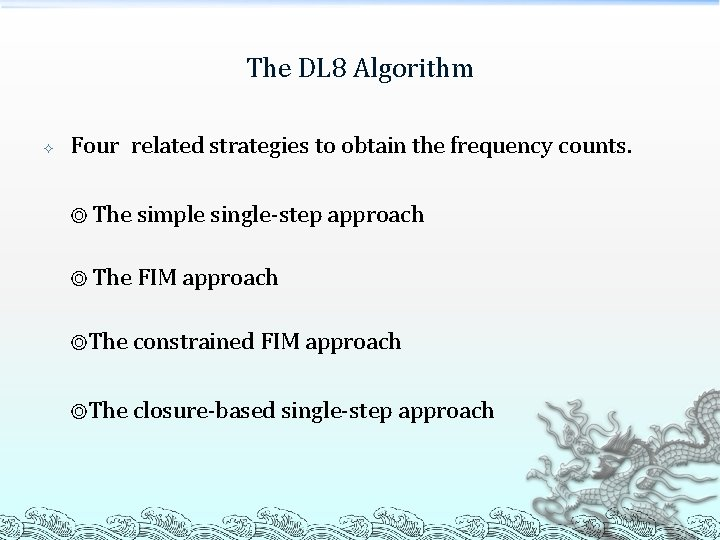The DL 8 Algorithm Four related strategies to obtain the frequency counts. ◎ The simple single-step approach ◎ The FIM approach ◎The constrained FIM approach ◎The closure-based single-step approachThe Simple Single-Step Approach DL 8 -SIMPLE The most straightforward approach Once DL 8 -RECURSIVE is called for an itemset I , we obtain the frequencies of I in a scan over the data, and store the result to avoid later recomputations.The FIM Approach Apriori-Freq+DL 8 Every itemset that occurs in a tree , must satisfy the local constraint p. Unfortunately, the frequent itemset mining approach may compute frequencies of itemsets that can never be part of a decision tree.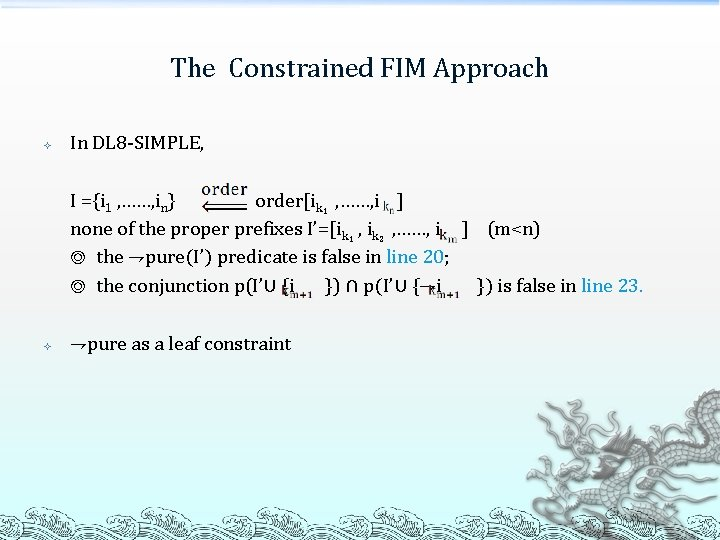The Constrained FIM Approach In DL 8 -SIMPLE, I ={i 1 , ……, in} order[ik₁ , ……, i ] none of the proper prefixes I’=[ik₁ , ik₂ , ……, i ] (m<n) ◎ the ⇁pure(I’) predicate is false in line 20; ◎ the conjunction p(I’∪ {i }) ∩ p(I’∪ {⇁i }) is false in line 23. ⇁pure as a leaf constraint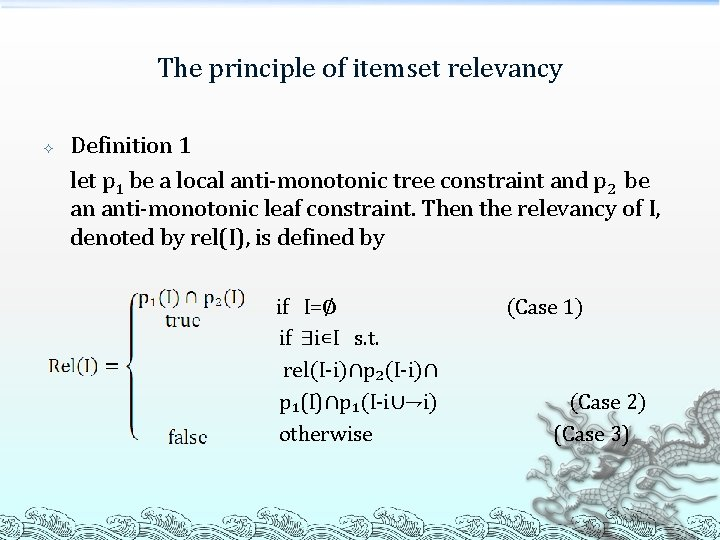The principle of itemset relevancy Definition 1 let p 1 be a local anti-monotonic tree constraint and p 2 be an anti-monotonic leaf constraint. Then the relevancy of I, denoted by rel(I), is defined by if I=∅ if ∃i∊I s. t. rel(I-i)∩p₂(I-i)∩ p₁(I)∩p₁(I-i∪⇁i) otherwise (Case 1) (Case 2) (Case 3)The principle of itemset relevancy Theorem 1 let L₁ be the set of itemsets stored by DL 8 -SIMPLE, and let L₂ be the set of itemsets {I⊆ I |rel(I)=true}. Then L₁=L₂. Theorem 2 itemset relevancy is an anti-monotonic property.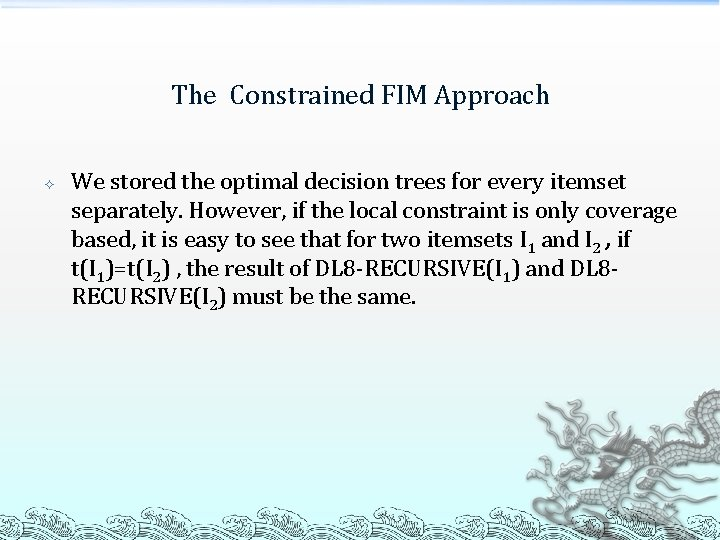The Constrained FIM Approach We stored the optimal decision trees for every itemset separately. However, if the local constraint is only coverage based, it is easy to see that for two itemsets I 1 and I 2 , if t(I 1)=t(I 2) , the result of DL 8 -RECURSIVE(I 1) and DL 8 RECURSIVE(I 2) must be the same.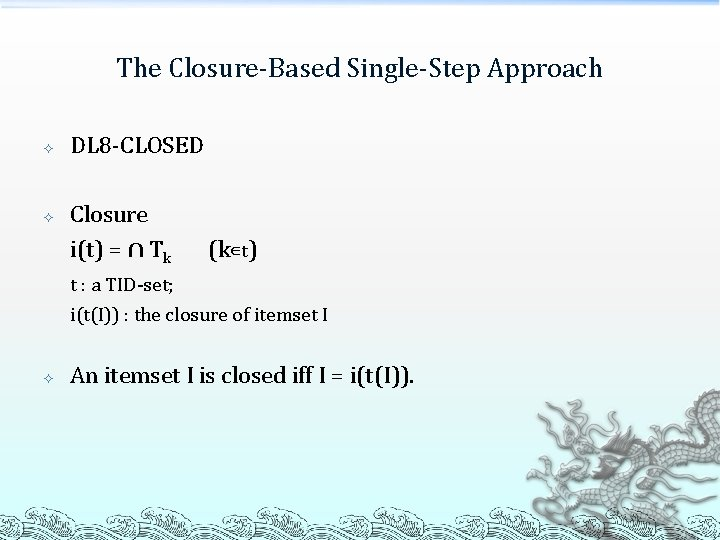The Closure-Based Single-Step Approach DL 8 -CLOSED Closure i(t) = ∩ Tk (k∊t) t : a TID-set; i(t(I)) : the closure of itemset I An itemset I is closed iff I = i(t(I)).Thank You !